# 魔幻滤镜Gabor Filter 原理与实现（python & C++）### Gabor Filter

• 复数形式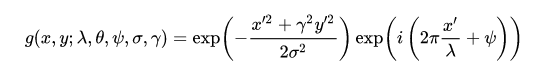• 实部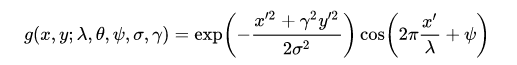• 虚部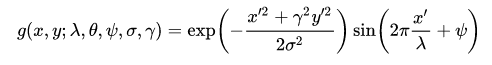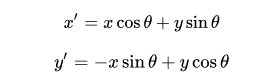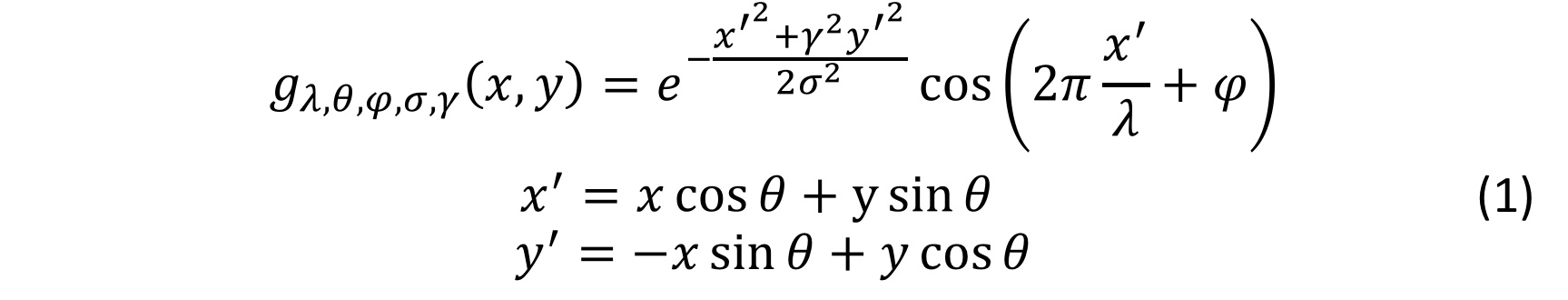A complex Gabor filter is defined as the product of a Gaussian kernel times a complex sinusoid.

Gaussian envelope mentiond in this method determines the width of the Gaussian kernel.

Paper— Fingerprint Image Enhancement: Algorithm and Performance Evaluation

### Python实现

import numpy as np

def gabor_fn(sigma, theta, Lambda, psi, gamma):
sigma_x = sigma
sigma_y = float(sigma) / gamma

# ------这部分内容是为了确定卷积核的大小------
# Bounding box
nstds = 3 # Number of standard deviation sigma
xmax = max(abs(nstds * sigma_x * np.cos(theta)), abs(nstds * sigma_y * np.sin(theta)))
xmax = np.ceil(max(1, xmax))
ymax = max(abs(nstds * sigma_x * np.sin(theta)), abs(nstds * sigma_y * np.cos(theta)))
ymax = np.ceil(max(1, ymax))
xmin = -xmax
ymin = -ymax
(y, x) = np.meshgrid(np.arange(ymin, ymax + 1), np.arange(xmin, xmax + 1))
# ------这部分内容是为了确定卷积核的大小------

# Rotation
x_theta = x * np.cos(theta) + y * np.sin(theta)
y_theta = -x * np.sin(theta) + y * np.cos(theta)

# ------这部分正是上面的公式（1）------
gb = np.exp(-.5 * (x_theta ** 2 / sigma_x ** 2 + y_theta ** 2 / sigma_y ** 2)) * np.cos(2 * np.pi / Lambda * x_theta + psi)
return gb

### Gabor函数的OpenCV实现

cv::Mat getGaborKernel(Size ksize, double sigma, double theta, double lambd, double gamma, double psi=CV_PI*0.5, int ktype=CV_64F );
cv2.getGaborKernel(ksize, sigma, theta, lambda, gamma, psi, ktype)

### 各参数含义

ksize：返回的滤波器的大小，即Gabor核的大小。如果ksize=(a，b)，那么Gabor核的大小即为axb像素。与许多其他的卷积核一样，这个尺寸一般取奇数而且为正方形。

sigmaσ是Gabor过滤器中实用的高斯函数的标准差。

thetaθ是Gabor函数的平行条纹的法线方向。有效值为从0~360度的实数。

lambdaλ是上述方程中正弦因子的波长。有效值应大于2，以像素为单位。

gammaγ是空间宽高比，表示Gabor滤波器的椭圆度

psiψ是相位偏移。有效值为-180度~180度，0度和180度对应的方程与原点对称，-90度和90度的方程分别于原点成中心对称，可两项直角坐标系中的余弦函数。

ktype：像素类型。

### OpenCV的Gabor源码

cv::Mat cv::getGaborKernel( Size ksize, double sigma, double theta,
double lambd, double gamma, double psi, int ktype )
{
double sigma_x = sigma;
double sigma_y = sigma/gamma;
int nstds = 3;
int xmin, xmax, ymin, ymax;
double c = cos(theta), s = sin(theta);

// ------这部分内容是为了确定卷积核的大小------
// ------可以看到，如果ksize不为0，则取ksize的一半------
if( ksize.width > 0 )
xmax = ksize.width/2;
// ------如果ksize为0，则按照维基百科的方式来取值------
else
xmax = cvRound(std::max(fabs(nstds*sigma_x*c), fabs(nstds*sigma_y*s)));

if( ksize.height > 0 )
ymax = ksize.height/2;
else
ymax = cvRound(std::max(fabs(nstds*sigma_x*s), fabs(nstds*sigma_y*c)));

xmin = -xmax;
ymin = -ymax;
//------这部分内容是为了确定卷积核的大小------

CV_Assert( ktype == CV_32F || ktype == CV_64F );

Mat kernel(ymax - ymin + 1, xmax - xmin + 1, ktype);
double scale = 1;
double ex = -0.5/(sigma_x*sigma_x);
double ey = -0.5/(sigma_y*sigma_y);
double cscale = CV_PI*2/lambd;

for( int y = ymin; y <= ymax; y++ )
for( int x = xmin; x <= xmax; x++ )
{
double xr = x*c + y*s;
double yr = -x*s + y*c;

// ------此处正是上面的公式（1）------
double v = scale*std::exp(ex*xr*xr + ey*yr*yr)*cos(cscale*xr + psi);
if( ktype == CV_32F )
kernel.at<float>(ymax - y, xmax - x) = (float)v;
else
kernel.at<double>(ymax - y, xmax - x) = v;
}

return kernel;
}

### 参考

##### 公众号CVPy，分享不仅限于OpenCV和Python的有意思的内容。02-121万+08-284638
08-163389
11-273864
07-273688
12-311534
01-02
06-02838
03-251346
12-051万+
05-041376
06-092627
09-081万+
11-23845
04-17241
03-121899
10-095万+